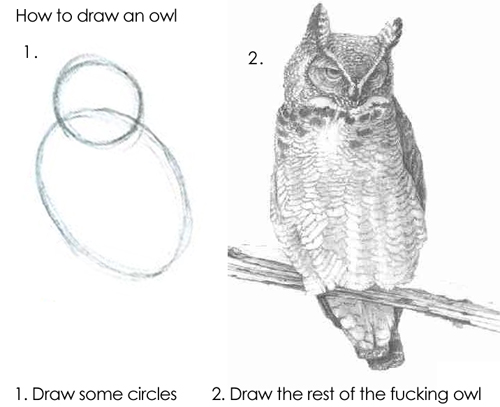# The Zerofox Syndicate

## A Canvas Background Animation

2020-05-22

Here is some code for a simple background animation created with 2d canvas.

## How to create an animated background

First add this piece of HTML.

``````<canvas id="bg"></canvas>
``````

Then write the rest of the required Javascript.

``````const canvas = document.getElementById("bg");
const c = canvas.getContext("2d");
const connDistance = 150;
const maxSpeed = 0.20;
const dotColor = "#444";
const dotDensityFactor = 30; // about the nr dots for a 720 screen

let dotAmount;
let dots = [];

function init() {
canvas.style = "position:fixed;top:0;left:0;width:100%;height:100%;" +
"z-index:-10;"
canvas.width = window.innerWidth;
canvas.height = window.innerHeight;
dotAmount = Math.floor((window.innerWidth * window.innerHeight / 855000)
* dotDensityFactor)
dots = Array.apply(null, Array(dotAmount)).map(function() {
return [
(maxSpeed * 2 * Math.random()) - maxSpeed,
(maxSpeed * 2 * Math.random()) - maxSpeed
];
});
}

function resize() {
canvas.width = window.innerWidth;
canvas.height = window.innerHeight;
dotAmount = Math.floor((window.innerWidth * window.innerHeight / 855000)
* dotDensityFactor)

if (dots.length < dotAmount) {
const diff = dotAmount - dots.length;
dots = dots.concat(Array.apply(null, Array(diff)).map(function() {
return [
// x
// y
(maxSpeed * 2 * Math.random()) - maxSpeed,
(maxSpeed * 2 * Math.random()) - maxSpeed
];
})
);
} else {
dots.length = dotAmount
}
}

function getDistance(p1, p2) {
// C² = A² + B²
return Math.sqrt(Math.pow(Math.abs(p1 - p2), 2) +
Math.pow(Math.abs(p1 - p2), 2));
}

function animate() {
requestAnimationFrame(animate);
c.clearRect(0, 0, innerWidth, innerHeight);
dots.forEach((d, i, arr) => {
c.beginPath();
c.arc(d, d, radius, Math.PI * 2, false);
c.strokeStyle = 'rgba(0, 0, 0, 0)';
c.fillStyle = dotColor;
c.fill();
c.stroke();
arr.forEach(d2 => {
const distance = getDistance(d, d2);
if (distance < connDistance && distance > 1) {
c.beginPath();
c.moveTo(d, d);
c.lineTo(d2, d2);
c.lineWidth = 8;
c.strokeStyle = dotColor;
c.stroke();

c.beginPath();
c.moveTo(d, d);
c.lineTo(d2, d2);
c.lineWidth = 2;
c.strokeStyle = 'rgba(255, 255, 255, 1)';
c.stroke();
}
});
});

dots.forEach((d, i, arr) => {
arr[i] += d;

// Handle border collisions
if (arr[i] < (0 + radius)) { arr[i] = Math.abs(d) }

if (arr[i] < (0 + radius)) { arr[i] = Math.abs(d) }

if (arr[i] > (innerWidth - radius)) {
arr[i] = 0 - Math.abs(d)
}

if (arr[i] > (innerHeight - radius)) {
arr[i] = 0 - Math.abs(d)
}
})
}